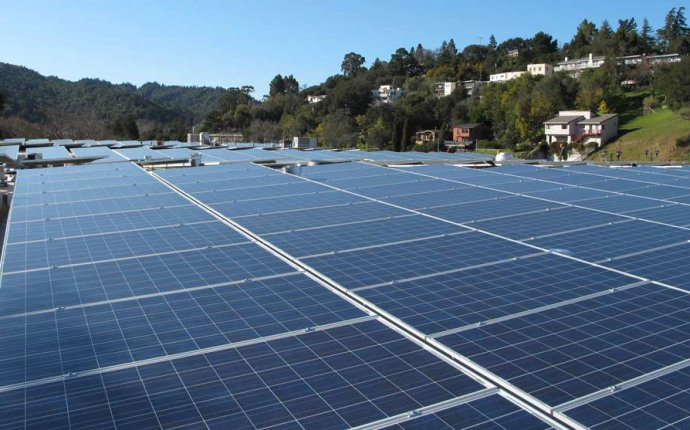## Power solar System

Solar Power / July 31, 2016Ever wonder what you can power with a 100W solar panel? Over 100, 000 viewers have…and now know the answer!

Watch below as we explore:

• How much power a 100W solar panel will generate
• What size battery and charge controller you need to store the power
• Ideas of what you can power with it…and for how long

Full Transcript

We sell a lot of solar panels for DIY off-grid solar projects. Generally, when we design a solar power system, we start with your loads, what you are trying to power, and from there you figure out what size solar panel you need. But now, we are going to look at it the other way around, what can you power with a 100W solar panel? A solar panel is rated by the amount of power it creates at Standard Test Conditions, or STC. These conditions include the intensity of the sun, 1000 watt per square meter, the angle of the light hitting the panel directly, the temperature, 25℃ or 77℉, and other criteria. So as they say, actual mileage may vary, based on all of these factors in the real world.

We generally reduce the calculations based on the difference between the lab setting and your actual installation. When a 12V solar panel is rated at 100W, that is an instantaneous rating, if all of the test conditions are met, when you measure the output, the voltage will be about 18 volts and the current will be 5.55 amps. Since watts equals volts times amps, 18 volts x 5.55 amps = 100 watts. Watts is like the speed of a car, miles per hour, how fast is it going at that instant, 50 miles per hour. To figure out how much power is generated over a period of time, you can to multiply the watts times the number of hours it is running. So in one hour, 100W x 1 hour = 100 watt hours. Again, with your car, 50 miles per hour x one hour equals 50 miles.

Now that we know the math behind it, we need to figure out how many hours to plug into the equation to determine how much power the solar panel will generate in a day. How many hours of sunlight that is equal to the intensity of standard test conditions, which is basically the sun at noon, will the solar panel be exposed to during the day? The number of hours of sunlight equal to noon is called insolation, or sun hours. As you well know, even though the sun is up at 8 in the morning, it is not as bright as it is at noon. So you can’t just say that the sun is shining for 10 hours, so I’ll multiple 100W x 10 hours. The hour between 8 and 9 in the morning is probably only half as strong as the sun from noon to 1 in the afternoon, so the morning hour would probably only be equal to ½ sun hour. But the days are so much shorter in the winter than the summer, the number of sun hours would be dramatically different throughout the year. Also, the amount of sunlight I’d get in MiamI, FL would be different than the amount of sun hours I’d get in Portland, ME.

Source: www.altestore.com